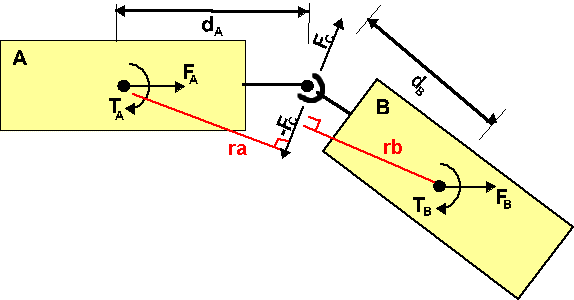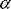# Physics - Jointed structures

This allows two nodes to be attached to each other in a flexible way so that forces in the plane of the joint will be transmitted through it, but forces perpendicular to the joint will cause it to bend. This will provide IK like capabilities.This can provide functionality from a simple hinged door, to a lattice framework, to a human body (loosely connected set of bones)

The idea is to build a program that will allow us to build virtual devices that can be built from standard components like Meccano or Lego.

### Types of Joint

In the field of robotics (or cybernetics), six basic types of joint have been defined:

 Name Symbol DOF Revolute joints R 1 Prismatic joints P 1 Helical joints - 1 Cylindrical joints RP 2 Spherical joints 3R 3 Planar joints RRP 3

In character animation, only 2 types of joint need to be considered. These are the "revolute" and "prismatic" joints. All other types can be based on these two.

#### 1 degree of freedom:

rotational joint - wheel.

hinge - similar to rotational joint above but with limits to motion (end stops)

#### 2 degrees of freedom

ball & socket joint

### Mathmatics of joints in dynamics systems

Joints might be thought of as a set of constraints on the dynamics equations describing the individual masses which are joined.

These constraints can be represented by equations.

One analytical solution would be to substitute the constraint equation into the dynamics equations. However this can lead to some complicated equations.

Another method to get a solution could be to use the method of Lagrange Multipliers.

Alternativly numerical methods could be used to solve the dynamics equations, with the constraints vetoing any movements not allowed by the joint.

### Solving for the motion of masses connected by a hinge using Lagrange Multipliers

Assume two masses, A and B, connected with a hinge. The only external forces acting on the system are:

• Fa = external force acting on the centre of mass of mass A.
• Fb = external force acting on the centre of mass of mass B.

And two torques acting on the system:

• Ta = external torque acting around the centre of mass of mass A.
• Tb = external torque acting around the centre of mass of mass B.There are no other external forces acting on the system. A force may be transmitted between the two masses Fc (by Newtons third law, the force of b of a is equal and opposite to the force of a on b. There is no torque between the two masses since a hinge is used.

Therefore the dynamics equations are:

{Fa} + {Fc} = ma * {aa}

{Fb} - {Fc} = mb * {ab}

 where: other definitions symbol description type units {Fa} external force on mass 'a' vector N=newton=kg*m/s2 {Fb} external force on mass 'b' vector N=newton=kg*m/s2 {Fc} force transmitted between hinge vector N=newton=kg*m/s2 ma mass of 'a' scalar kg {aa} linier acceleration of 'a' vector m/s2 mb mass of 'b' scalar kg {ab} linier acceleration of 'b' vector m/s2

Newtons second law, applied to angular momentum, the net extenal torque acting on a system equals the rate of change of the angular momentum of the system. Angular momentum L=Iw and T=I.

Assume we are working in the frame of reference of object 'a'. Therefore we can use the following equations (from dynamics 1 - case 2)

Tax = Iaxx aax
Tay = Iayy aay
Taz = Iazz aaz

Since object 'b' may be moving with respect to object 'a' then we need to use dynamics 1 - case 3:

Tbx = Ibxx abx - Ibxy (aby - wbx wby) - Ibxz (abz + wbx wby) - (Ibyy - Ibzz) wby wbz - Ibyz (wby wby - wbz wbz)
Tby = Ibyy aby - Ibyz (abz - wbx wby) - Ibxy (abx + wby wbz) - (Ibzz - Ibxx) wbx wbz - Ibxz (wbz wbz - wbx wbx)
Tbz = Ibzz abz - Ibxz (abx - wby wbz) - Ibyz (aby + wbx wbz) - (Ibxx - Ibyy) wbx wby - Ibxy (wbx wbx - wby wby)

 where: other definitions symbol description type units Ta external torque on a about centre of mass bivector Nm=kg*m2/s2 Tb external torque on b about centre of mass bivector Nm=kg*m2/s2 Ia Inertia on a about centre of mass of a matrix kg*m2 Ib Inertia on b about centre of mass of b matrix kg*m2a angular acceleration of a bivector s-2b angular acceleration of b bivector s-2 ra perpendicular offset between the reaction force and centre of mass of a. vector m rb perpendicular offset between the reaction force and centre of mass of b. vector m

This can be written in matrix form as:

{Tb} = [Ib] {b} + f(Ib,wb)

 The constraints on the system due to the hinge is: sq(Pya) + sq(Pxa) = sq(da) sq(Pyb) + sq(Pxb) = sq(db) Where Pya = position of centre of mass a in y coordinate relative to hinge. Pxa = position of centre of mass a in x coordinate relative to hinge. Pyb = position of centre of mass b in y coordinate relative to hinge. Pxb = position of centre of mass b in x coordinate relative to hinge. da = distance of centre of mass a from hinge db = distance of centre of mass b from hinge   Fc is an unknown, if we could find the value of fc then the solution would be easy. Also ra and rb are unknown (because the direction of Fc is unknown). Therefore to slimplify the geometry, we could translate to rotations about the hinge. To do this we can use the parallel-axis theorm: I = Icm + M*sq(h).

 metadata block see also: go on to deformations Correspondence about this page Open Forum discussion - Joint Constraints Book Shop - Further reading. Where I can, I have put links to Amazon for books that are relevant to the subject, click on the appropriate country flag to get more details of the book or to buy it from them.Robots and Screw Theory: Applications of Kinematics and Statics to Robotics (Hardcover) - This is about the mechanics of robot arms such as those used such as those used in factory automation. Assumes a good knowledge of mechanics but the concepts of robot arms and screw theory are introduced gradually. The maths used is fairly conventional: scalars, vectors and matrices. Dual quaternions (or biquaternions as they call them) are only mentioned briefly. Commercial Software Shop Where I can, I have put links to Amazon for commercial software, not directly related to the software project, but related to the subject being discussed, click on the appropriate country flag to get more details of the software or to buy it from them.LEGO Mindstorms NXT - Allows you to build a robot and program it from a PC or Mac. Contains an Intelligent Brick with 32-bit microprocessor, memory and FLASH, 3 Interactive Servo motors with built-in rotation sensors, sound sensor, ultrasonic sensor, touch sensor, light sensor, USB 2.0 and Bluetooth support. Icon-based drag-and-drop program "building" environment.

This site may have errors. Don't use for critical systems.